**Lessons Learned and Improvements when Building Screen-Space Samplers with Blue-Noise Error Distribution** ***Supplemental material*** Convergence rates ======================================================================================= Here, we compare the convergence rates of the Sobol sequence with Owen scambling compared to the Rank-1 Lattice. We used those sequence the same way we use them in the screen-space sampler (with scrambling and padding). The Sobol sequence uses XOR scrambling (that preserves convergence) and the Rank-1 sequence uses Cranley-Patterson Rotations
Convergence for :!!! Tip **In summary**, we found that while the Sobol sequence with Owen scrambling ensures superior convergence properties (thanks to multi-stratification), **in practice Rank-1 Lattice perform as par on rendering integrands** after dimension padding and per pixel scrambling. Implementation ======================================================================================= Our new screen-space sampler is still easy to implement in a modern rendering engine. It requires a few lines of code to work: ~~~~~~~~~~~~~~~~~~~~~~~~~~~~~~~~~~~ C // Return the k-th sample for the d-th dimension of the sequence // at pixel p of the tile. Assumes that pixel coordinates, sampling // count and dimensions are wrapped to the tile dimensions. // float sample(int2 p, int k, int d) { float shift = m_tile(p.x, p.y)[d]; return mod(m_direction[d]*k + shift, 1.0) } ~~~~~~~~~~~~~~~~~~~~~~~~~~~~~~~~~~~ Blur Criterion on Test Integrands ======================================================================================= To assess the quality of this new screen-space sampler, we developped an quantifiable criterion in order to make a rational decision. This quantifiable criterion is the average variance of tiles of the test integrands (randomly oriented heavisides). It is defined as: $$\mbox{RMSE} = \mbox{Var}[ k_\sigma \circledast e^{p}_t ]$$ where $e^{p}_t = {1\over N} \sum_k f_t(\mathbf{s}_p^k) - \int f_t(\mathbf{x}) \mbox{d}\mathbf{x}$ is the tile of the Monte-Carlo estimation of the test integrand $f_t(\mathbf{x})$ for each pixel $p$ of the tile.
Convergence atFor small sample counts, the two sequences are reducing the RMSE with roughly the same slope. However, as we increase the number of samples, the Rank-1 based screen-space sampler reduce the variance faster than the Sobol with Owen scrambling one. Blur Criterion on Rendering Scenes ======================================================================================= We validate that the objectivable criterion on the test integrand is correlated with the denoising quality for actual renderings. Here we report the RMSE of our screen-space samplers after a screen space blur of varying size: $$\mbox{RMSE} = \left\lVert \left( k_\sigma \circledast I_{{ref}} \right) - \left( k_\sigma \circledast I \right) \right\rVert_2,$$ where $I$ is the rendered image with our screen-space sampler, $I_{ref}$ is the reference image, and $k_\sigma$ is a Gaussian blur kernel of standard deviation $\sigma$. If the rendering noise has localy a constant amplitude, using a sampler that distribute the error as a blue-noise will ensure a reduced RMSE for various kernel sizes.
Convergence for at!!! Tip **In summary**, we observe that the results of computing the RMSE on rendering integrands follows the same behaviour as for the test integrands. !!! Warning You can find a side-by-side comparison to visually validate that this criterion produces better looking images as well here. Eigenvectors of an Optimization ======================================================================================= The optimization loss is defined from the L2-norm of the matrix of error. Since this matrix of error is build on the vectors of estimates, it lies in a N-dimensional vector space. Changing the main sequence will change the values in the vectors of estimates, and results in a different vector space. We extracted the eigenvectors $\mathbf{v}^k$ and eigenvalues $\lambda^k$ of such vector space for different main sequences (independent, Sobol with Owen scrambling and Rank-1). Each eigenvector defines an eigenintegrand: $$g_k(\mathbf{x}) = \sum_t v^k_t f_t(\mathbf{x}),$$ and the associated eigenvalue, tells us how much this integrand is important for the optimization. This link shed some light regarding the noise distribution of some sampler. For example, in the following we display the eigenvectors resulting from the optimization of 1024 test integrands at 16 spp. We display the first 3 eigenvectors with the cumulative sum of absolute eigenvalues $\mbox{cum}_e$. For an indepdendant sampler (where each sample is obtained from a random number generator) we obtain the following eigenvectors:$c_1 = 0.299$$c_2 = 0.595$$c_3 = 0.650$$c_4 = 0.702$$c_5 = 0.740$
For our previous screen-space sampler with a Sobol sequence with Owen scrambling as main sequence we obtain the following eigenvectors: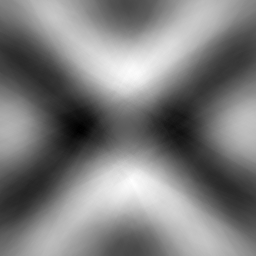$c_1 = 0.217$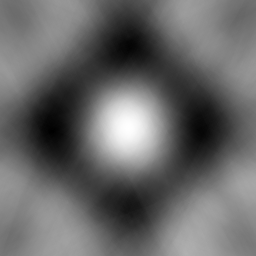$c_2 = 0.402$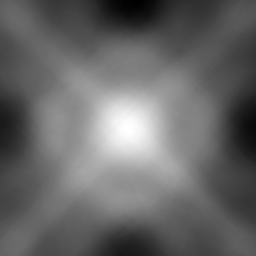$c_3 = 0.450$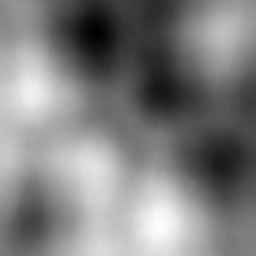$c_4 = 0.508$$c_5 = 0.523$
Using our improved screen-space sampler with Rank-1 main sequence we obtain the following eigenvectors:$c_1 = 0.219$$c_2 = 0.379$$c_3 = 0.463$$c_4 = 0.544$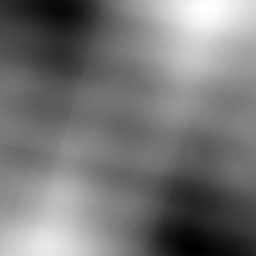$c_5 = 0.606$
!!! Tip **In summary**, we observe that the main sequence we used previously [Heitz et al. 2019] produces eigenintegrands with higher-frequencies than the one of the independent sampler and the Rank-1 main sequence. We conjecture that this is linked with the quality of the error distribution performances of the screen-space sampler.